## General Mechanical

•jackhero
Subscriber

I followed the discussion on Three point bending to design my model for static structural non-linear three point bending simulation with symmetry. The block size is 160x40x40 mm with a span length of 120 mm.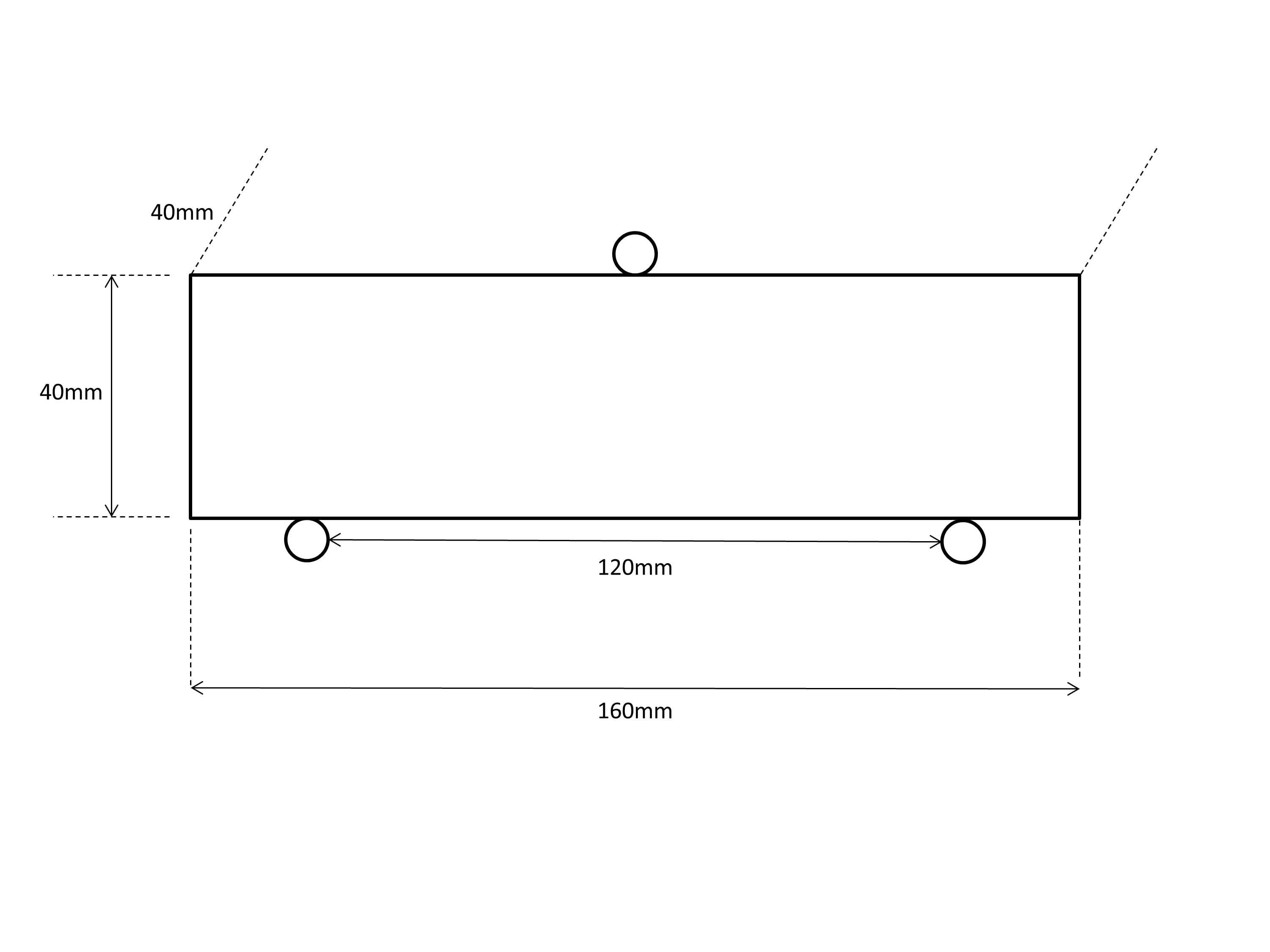For symmetry, I reduced the model to 160/2 mm (in length) and 40/2 mm (in width). I ran the model but I think I am mistaking somewhere either in symmetry or in boundary conditions. I would like to request guidance on the following queries;

(1). Originally in the complete 3D design (without symmetry) I split the face to make place for the rollers (both top and bottom each with 10 mm of face split length). If I reduce the model length wise i.e. 160/2mm, I also reduced the face split of the top roller to 5 mm. Is it correct?

(2). When I model symmetry, in the present case is the quarter model, I should apply the boundary condition load (displacement controlled) 1/4th of the original value. Will the resultant values (for force, deformation, stress, strain etc) will only be for the 1/4th of the model or for complete model?. I need to compare the experimental results with that of the simulation.

(3). I created the symmetry as following;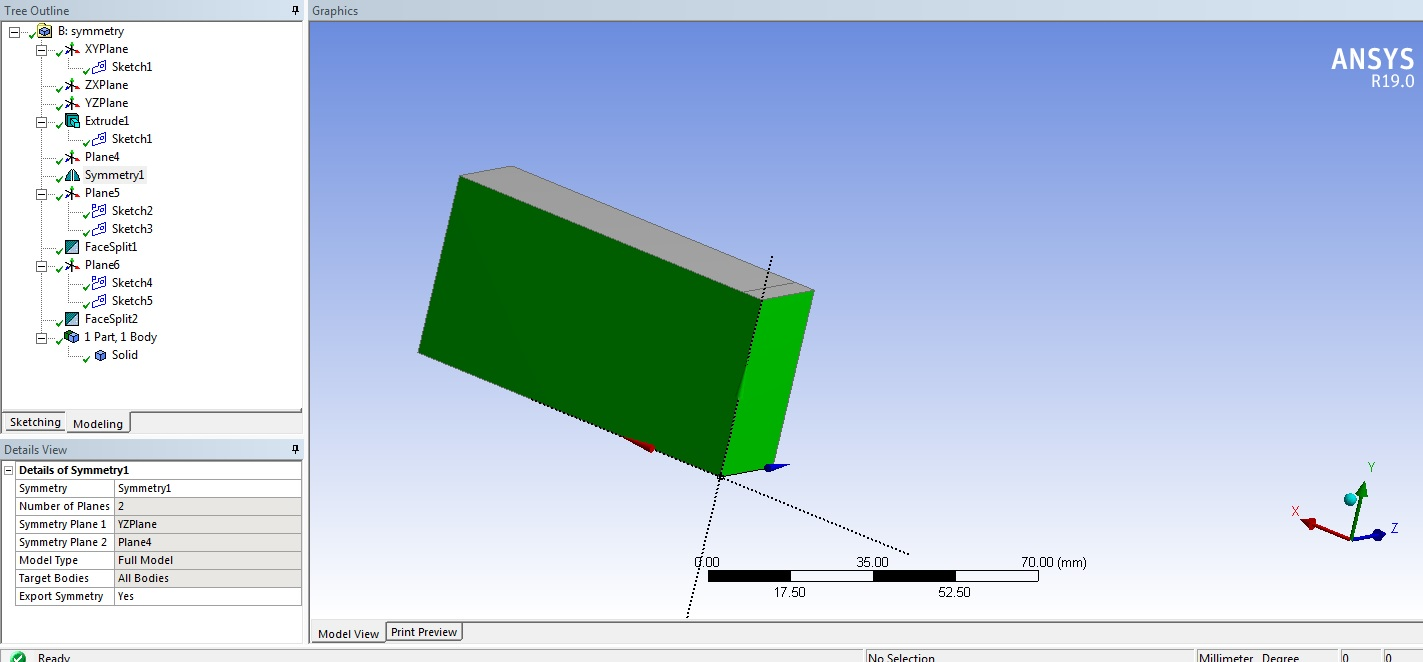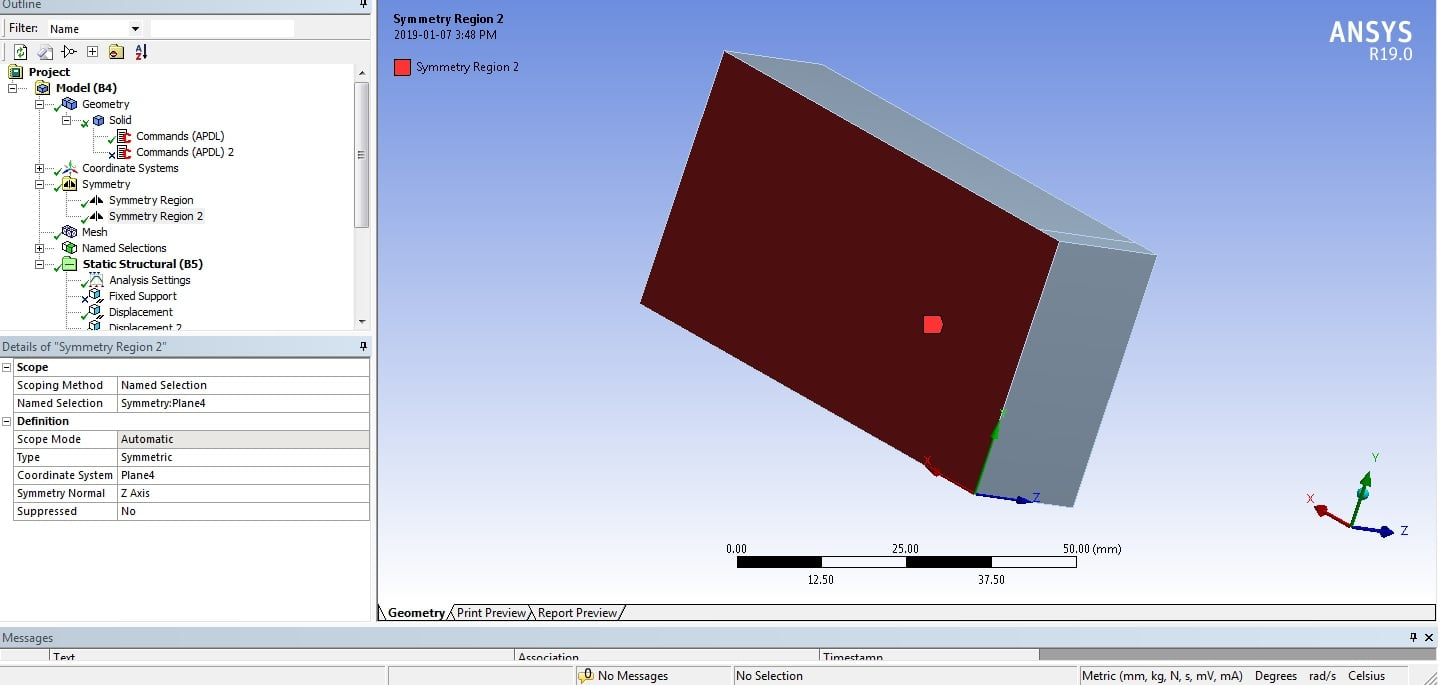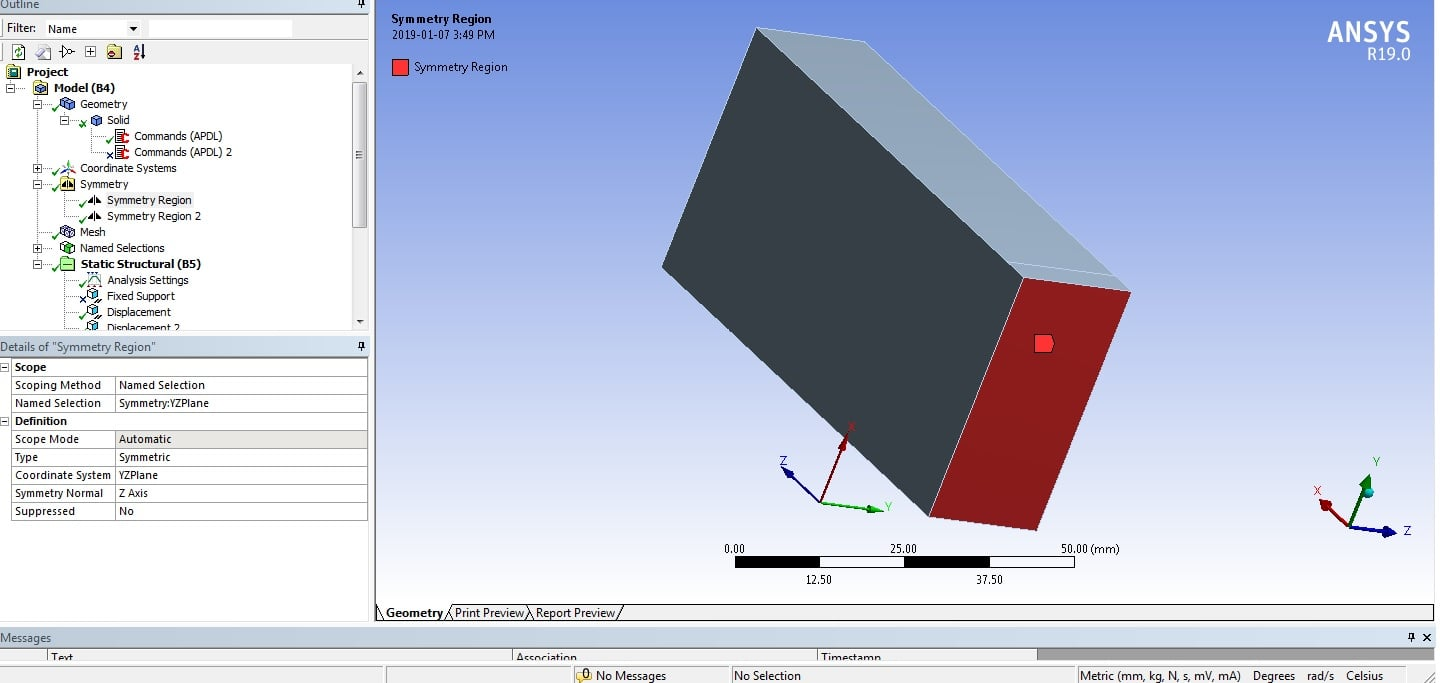Is the symmetry designed above is correct? One of the post here suggested to make symmetry on both faces and edges. What will be the difference if I only make symmetry on the faces only as I have done above?

(4). I applied the boundary conditions as following; with displacement A as x=free, y=-0.5mm, z=free. And displacement B as x=free, y=0mm, z=0mm. Could someone please correct me on applying the proper boundary conditions?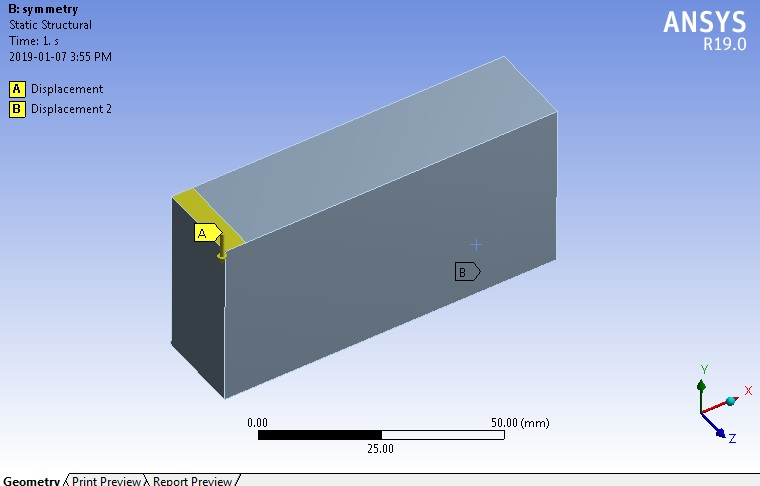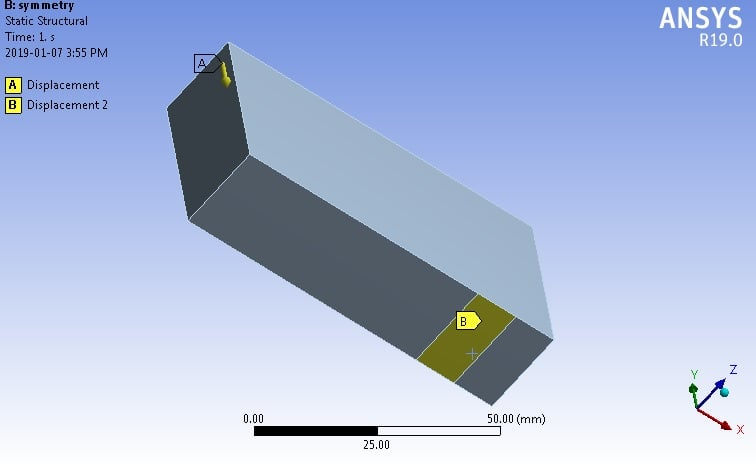With in the discussion the remote (loading) displacement was suggested. I am not clear about on how the rotations and components are decided to be set free or set as constant? Also, since I will be modeling the non-linear behavior so I shall keep the remote displacement behavior as deformable?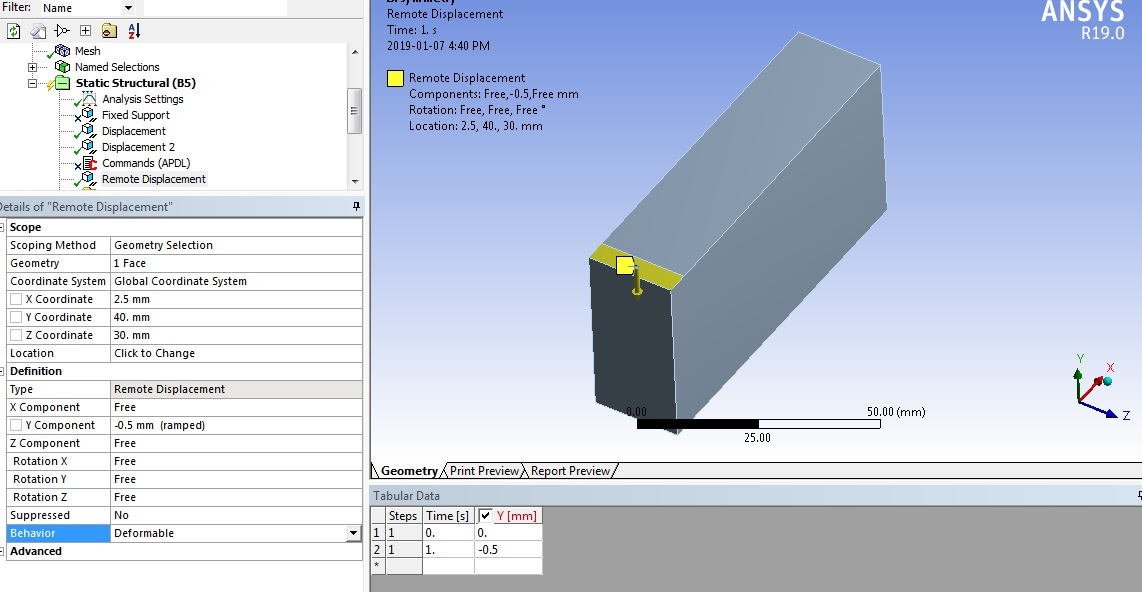•jj77
Subscriber

1. I would start with half symmetry just to understand things (but without thinking to much, it should be OK).

2. In half symmetry, the load needed for the half sym. model to get same displacements as in the full model is half of the full model. Thus if your load on the full model is 1 N, then apply 1/2 N in the half. symm. model. In the same logic a quarter model, needs only 1/4 N.

If you use displacement control and apply enforced displacements in the half symm. model.  then just apply the displacement incrementally, and when you look on the reaction there to get the equivalent applied force, you must double that reaction force to get the correct value as in the full model, and in order to compare to exp..

3. I never use any default symmetry. It is important to understand symm. and how it works, so I put enforced displacements myself. Along the length your need to fix X, and across the width Z.

4. for the bottom rollers, I would represent them, and use a contact in between them, and the beam, since the area that is fixed/incontact, changes as the beam deflects. Thus it is a nonlinear contact situation not a linear standard permanent fixed boundary. As such you need to use the nonlinear solver for the contact ,and perhaps any large deflections and plasticity if you have that.

•peteroznewman
Subscriber

Hello Jack,

(1) Yes, you would end up with half the width of the center load pad when you cut the model in half at the center.

(2) As jj77 said above, you apply the full displacement distance in -y that you need to get to a failure and add a Reaction Force Probe, pointing to the Remote Displacement, to look at the force along the way. You multiply by 4 the force from the 1/4 model to recover the force a full model would have given and to compare with experimental data.

(3) The symmetry is correct. When you pick a face, the nodes on the edge of the face are included, you don't have to pick the edge geometry as well as the face. I trust ANSYS to do the constraints properly, but I understand jj77's approach of defining the constraint explicitly.

(4) The problem with displacement on a face is the entire support face has to remain flat. This is an overconstraint compared with the real beam which has an angle change, especially at the end support. The Remote Displacement replaces the support face with a point and now the point can have Y=0 (at the end) and all other DOF remain Free so that the support face can have an angle change. The point will be created at the centroid of the face, but you can move it if you want in the Details window. You must move the point on the top load face from the centroid to the symmetry planes. Then the arrow will appear on a corner of the 1/4 model, but the load will be distributed to the top load face. There will probably be little difference in the results between Rigid or Deformable behavior for the Remote Displacement. Start with Deformable and look at the results.

The benefit of Remote Displacement over contact is that RD is a linear constraint, meaning that the solver can compute its solution in one iteration, while contact is a nonlinear constraint and the solver must use multiple iterations to compute the solution. You have material and geometric (Large Deflection=On) nonlinearity in the model, so the solver will do multiple iterations anyway. There may not be a large difference in solution time, but you have to do more geometry prep for contact, since you need to create the surface on which to make contact, and then add support to that surface and deal with potential convergence problems in the contact.

If the supports are at a fixed span, contact is more accurate as jj77 explains, because the beam will pull inward as the center fails, and the contact point on the beam will slide closer to the end as the beam pulls inward. The RD point will move inward with the beam, which is a small error in the model compared with the real model, but this is a second order effect for small -y center displacements. I prefer to use a simpler model and get a result with the first order effects, then add complexity to look at second order effects in a second model.

Regards,
Peter

•jackhero
Subscriber

Thank you jj77 and Peter for your guidance. Both of the replies are helpful and informative.

One last query before I mark the solution. I have kept the remote displacement for the support at the default centroid of the face at the bottom.

You must move the point on the top load face from the centroid to the symmetry planes. Then the arrow will appear on a corner of the 1/4 model, but the load will be distributed to the top load face.

But for the top face the default position of the remote displacement was as following,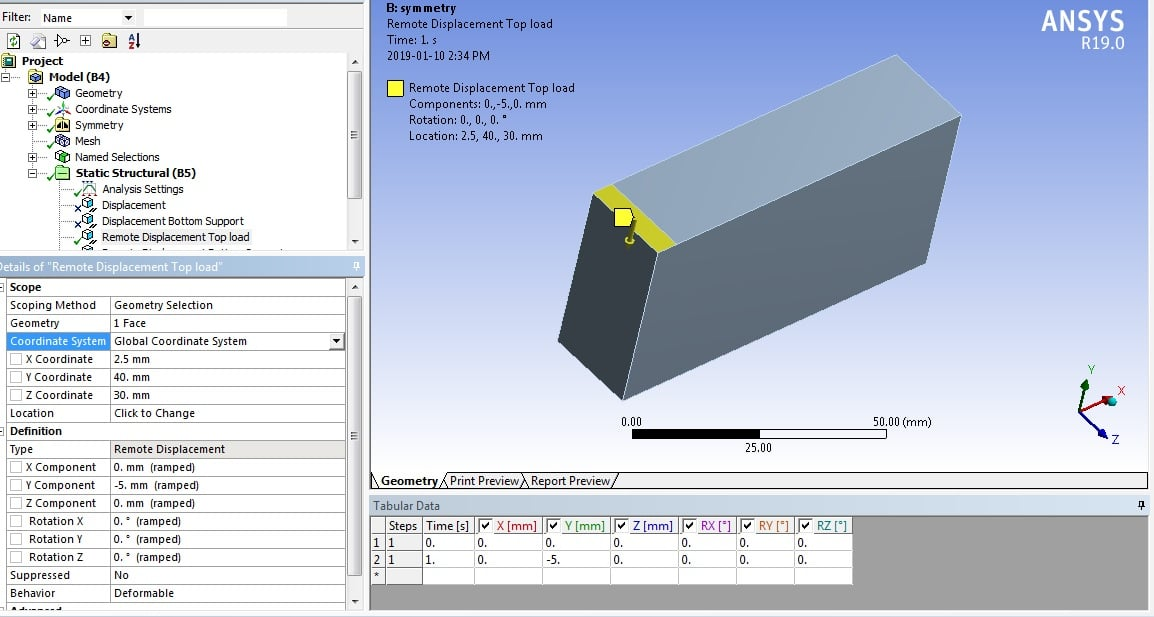I selected one of the symmetry planes (total I have two symmetry planes) and I had the remote displacement being shown as the following,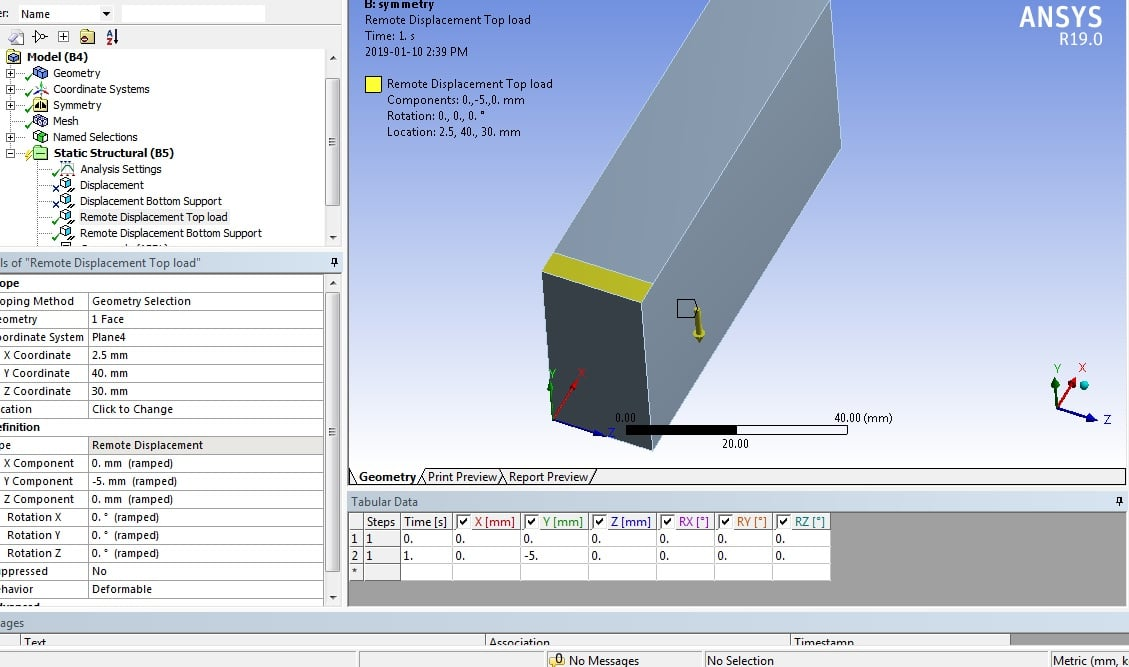According to the guidance, the point shall appear on a corner of the 1/4 model. But for me the point is being shown outside the 1/4 model. How shall I adjust it manually or it is at the correct position already?

•peteroznewman
Subscriber

You should adjust it manually. If you set the geometry filter to Vertex, you can click on the corner of the body at the center planes. On the bottom of the Mechanical window you will see the x,y,z coordinates of that vertex. Go to the Remote Displacement, in the Details window edit the Coordinates to the correct values.

Regards,
Peter